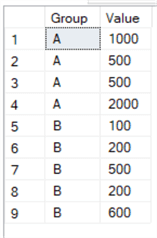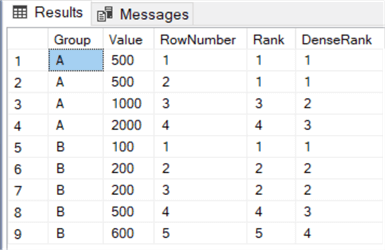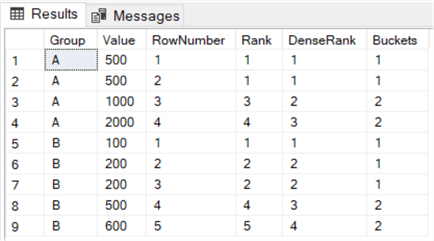# SQL Server Window Functions RANK, DENSE_RANK and NTILE

##### Overview

The topic of this part is about ranking functions. They were introduced in SQL Server 2005. ROW_NUMBER is also a ranking function, but it has already been covered extensively in the previous part of this tutorial.

## RANK and DENSE_RANK

In contrast with the ROW_NUMBER function, RANK and DENSE_RANK don’t have to generate unique numbers. The difference between all these functions is how they handle ties.

• ROW_NUMBER will always generate unique values without any gaps, even if there are ties.
• RANK can have gaps in its sequence and when values are the same, they get the same rank.
• DENSE_RANK also returns the same rank for ties, but doesn’t have any gaps in the sequence.

Let’s illustrate with an example. Suppose we have the following sample values:We can write the following query to test the three ranking functions:

```SELECT
[Group]
,[Value]
,RowNumber    = ROW_NUMBER() OVER (PARTITION BY [Group] ORDER BY [Value])
,[Rank]       = RANK()       OVER (PARTITION BY [Group] ORDER BY [Value])
,[DenseRank]  = DENSE_RANK() OVER (PARTITION BY [Group] ORDER BY [Value])
FROM [dbo].[Test];
```

This gives us this result set:As you can see, RANK will return the same rank for ties. However, the next rank in group A is the number 3, omitting rank 2. DENSE_RANK on the other hand doesn’t skip rank 2. When there are no ties in the record set, all three ranking functions return the exact same result.

With ROW_NUMBER and RANK, we can create an alternative construct to detect duplicate rows. With the same sample data used in part 5 of this tutorial, we can create the following query to find all rows that have a duplicate business key:

```SELECT
[EmployeeKey]
,[EmployeeName]
,[InsertDate]
,RID    = ROW_NUMBER()  OVER (ORDER BY [EmployeeKey])
,RankID = RANK()        OVER (ORDER BY [EmployeeKey])
FROM [dbo].[EmployeesDuplicate];
```

The result set:Now we only need to filter on records where the generated row number (RID) is different from the rank (RankID). This will return a record for Bob, so we know there’s a duplicate record for that employee.

#### When to use RANK or DENSE_RANK?

As specified before, RANK can have gaps in the sequence and DENSE_RANK doesn’t. Suppose you have the task to find the top 5 customers according to sales value. With RANK, you can rank your customers and then filter out any customers with a rank bigger than 5. If you would do the same with DENSE_RANK, you might end up with more than 6 customers!

Suppose we have this set of data about our customers:When adding ranks with RANK and DENSE_RANK, we have the following data set:When filtering on rank <= 5, you’ll get exactly 5 rows returned. However, if you filter on denserank <= 5, you’ll get 6 rows, which might not be what you want. The more ties, the more rows DENSE_RANK can return. If the tie would be on exactly row number 5, the RANK function would also return 6 rows. In that case, you need to “break the tie”. You can do this by using the ROW_NUMBER function or by sorting on additional column.

## NTILE

The last ranking function is a bit special. NTILE divides the rows in roughly equal sized buckets. Suppose you have 20 rows and you specify NTILE(2). This will give you 2 buckets with 10 rows each. When using NTILE(3), you get 2 buckets with 7 rows and 1 bucket with 6 rows.

Let’s reuse the query from the previous section, but add an expression using NTILE:

```SELECT
[Group]
,[Value]
,RowNumber    = ROW_NUMBER() OVER (PARTITION BY [Group] ORDER BY [Value])
,[Rank]       = RANK()       OVER (PARTITION BY [Group] ORDER BY [Value])
,[DenseRank]  = DENSE_RANK() OVER (PARTITION BY [Group] ORDER BY [Value])
,Buckets      = NTILE(2)     OVER (PARTITION BY [Group] ORDER BY [Value])
FROM [dbo].[Test];
```

We get the following results:An example where NTILE was used to divide data into buckets is described in the tip How to create a heat map graph in SQL Server Reporting Services 2016.